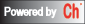## System Conversion

The type of a control system can be of continuous-time and discrete-time. Functions below can be used to convert system from continuous-time to discrete-time or vice versa, change the sampling time of a discrete-time system, or perform a state coordinate transformation for state-space models.

Function
`    `
Description
Coordinate transformation Change state coordinates for state-space models.
Continuous to discrete-time Convert system from continuous-time models to discrete-time models.
Discrete-time to continuous  Convert system from discrete-time models to continuous-time models.
Discrete to discrete model Create an equivalent discrete-time model with new sample time.
Delay2z Map all time delays to poles at z=0 for discrete-time system.